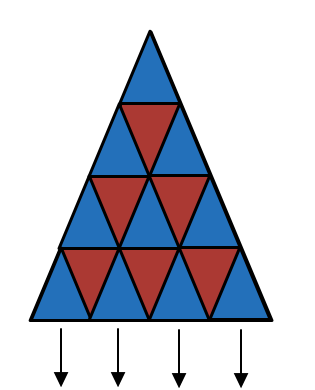# Extended Triangle-ception

Logic Level 4The diagram shows the tiling of the first $4^2$ smallest congruent equilateral triangles.

The extension of this triangle has a total of $n^2$ smallest congruent equilateral triangles.

Find the positive value of $n$ if the total number of triangles (of all sizes) for this diagram is 988.

×

Problem Loading...

Note Loading...

Set Loading...